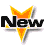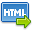Journal :   International Journal of Technology

Volume No. :   4

Issue No. :  1

Year :  2014

Pages :   197-202

ISSN Print :  2231-3907

ISSN Online :  2231-3915Registration

## Logarithmic mean Newton’s method for simple and multiple roots of Nonlinear Equations

 Address: K. L. Verma NSCBM Govt. Post Grdauate College, Hamirpur (HP) INDIA *Corresponding Author
DOI No:

ABSTRACT:
In this paper the convergence behavior of a variant of Newton’s method based on the Logarithmic mean for solving nonlinear equations is proposed. The convergence properties of this method for solving equations which have simple or multiple roots have been discussed and it is shown that it converges cubically to simple roots and linearly to multiple roots. Moreover, the values of the corresponding asymptotic error constants of convergence are determined. Theoretical results have been verified on the relevant numerical problems. A comparison of the efficiency of this method with other mean-based Newton’s methods, based on the others means, is also included.
KEYWORDS:
Newton’s method; Iterative methods; Mean-based Newton’s method; Logarithmic mean: Order of convergence; Asymptotic error constant.
Cite:
K. L. Verma. Logarithmic mean Newton’s method for simple and multiple roots of Nonlinear Equations. Int. J. Tech. 4(1): Jan.-June. 2014; Page 197-202[View HTML][View PDF]

Visitor's No. :   124732